# F(x)=(x+1)^0.5, h(x)=x^2+3, what is the range of f(h(x))?

• Darkmisc

#### Darkmisc

Homework Statement
f(x)=(x+1)^0.5, h(x)=x^2+3, what is the range of f(h(x))?
Relevant Equations
f(x)=(x+1)^0.5, h(x)=x^2+3
Hi everyone

I have solutions to this problem, but still don't understand them.

I thought the range of h(x) was [3, \infty). Wouldn't this make the domain of f(h(x)) also [3, infinity)?

If this is correct, shouldn't the range of f(h(x)) be [13^0.5, infinity)?

Below is a graph of f(h(x)) using the assumption that the range of h(x) is [3, infinity)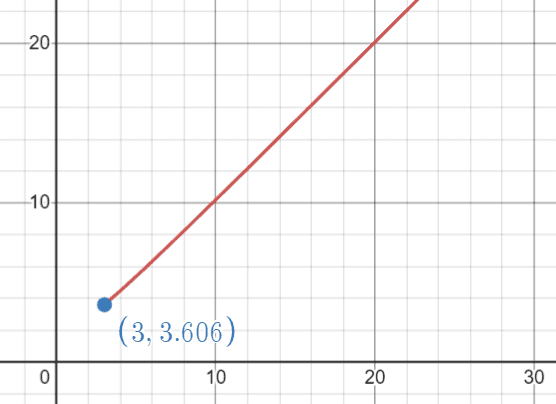The solutions say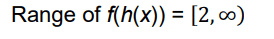But the domain of f(h(x)) would need to include 0 for this to be true.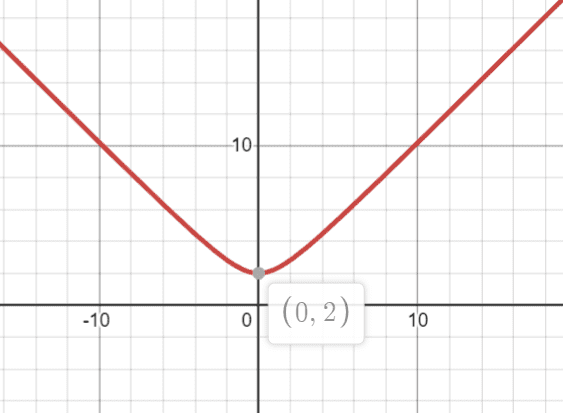Thanks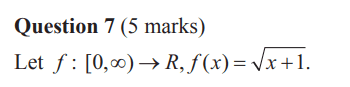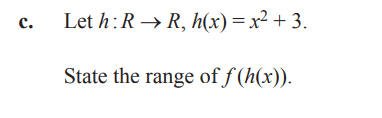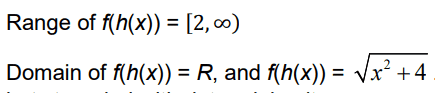#### Attachments

Homework Statement:: f(x)=(x+1)^0.5, h(x)=x^2+3, what is the range of f(h(x))?
Relevant Equations:: f(x)=(x+1)^0.5, h(x)=x^2+3

Hi everyone

I have solutions to this problem, but still don't understand them.

I thought the range of h(x) was [3, \infty). Wouldn't this make the domain of f(h(x)) also [3, infinity)?

If this is correct, shouldn't the range of f(h(x)) be [13^0.5, infinity)?

Below is a graph of f(h(x)) using the assumption that the range of h(x) is [3, infinity)

View attachment 316487

The solutions say View attachment 316489

But the domain of f(h(x)) would need to include 0 for this to be true.
View attachment 316490

Thanks

View attachment 316483
View attachment 316484

View attachment 316485
What is the range of h(x)? How does this compare with the domain of f(x)?

-Dan

•Darkmisc
What is the range of h(x)? How does this compare with the domain of f(x)?

-Dan
Thanks.

range of h(x) is [3, infinity).

If I take [3, infinity) as the domain of f(x), the range becomes [2, infinity), which is the answer from the solutions.

I got the wrong answer by using [3, infinity) as the domain of f(h(x)), although I don't really understand why it is wrong.

range of h(x) is [3, infinity).

If I take [3, infinity) as the domain of f(x), the range becomes [2, infinity), which is the answer from the solutions.
That's right, because ##[2, \infty)## is the range of ##h##.
I got the wrong answer by using [3, infinity) as the domain of f(h(x)), although I don't really understand why it is wrong.
It's wrong, because ##f(h(x))## is well-defined for all ##x \in \mathbb R##. Just write it down:
$$f(h(x)) = \sqrt{x^2 + 4}$$If I gave that function to a student and asked what was its domain, they wouldn't say ##[3, \infty)##. Why would they?

•topsquark and Darkmisc
I got the wrong answer by using [3, infinity) as the domain of f(h(x)), although I don't really understand why it is wrong.
You already took care of h(x) when you figured out that its range was ##[3,\infty)##. Now you want the range of f(z), NOT f(h(x)), for z in the domain of ##[3,\infty)##. Plugging 3 into f should give you 2.

•topsquark and Darkmisc